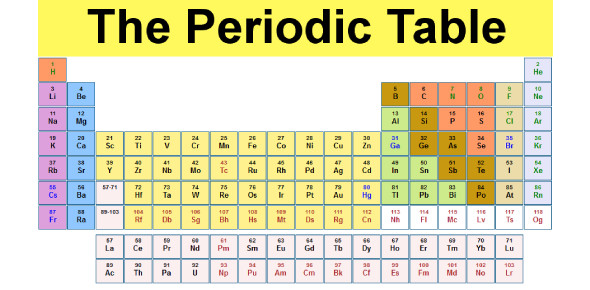# Quiz: The Periodic Table Trivia Questions

15 Questions | Total Attempts: 82Settings. Do you know anything about the periodic table? How do you think you would do on this quiz? The periodic chart of elements is a tabular exhibition of chemical elements that are organized by atomic number, electron structure, and recurrent chemical properties. The composition of the table shows periodic trends. Take this quiz and see how much you know about the periodic table.

• 1.
What does the symbol in the upper left-hand corner stand for?
• A.

Atomic Number

• B.

Mass of the Element

• C.

How many electrons

• 2.
Why is Hydrogen not grouped with any of the others?
• A.

Because it wants to

• B.

Because it doesn't share properties with any of the others

• C.

Because it has a unique atomic number

• 3.
Why did we color code the Periodic Table?
• A.

To make it look pretty

• B.

To show which elemts want to be friends

• C.

To show the different categories

• 4.
What are the three main categories?
• A.

Green,purple,and pink

• B.

Metalloid, metal, and non-metals

• C.

None are important this test is lame!

• 5.
Which is equivilant to the atomic number?
• A.

Protons

• B.

Orbits

• C.

Electrons

• 6.
How do you get the amount of neutrons in each element?
• A.

Subtract the electrons from the mass

• B.

• C.

Divide the mass by the protons

• 7.
What is the last column of elements?
• A.

Elements. duh

• B.

Noble gasses

• C.

• 8.
What does the largest grouped category represent?
• A.

Alkaline earth metals

• B.

Non-metals

• C.

Transition metals

• 9.
What do the elements in the first column (besides hydrogen) represent?
• A.

Alkali Metals

• B.

Antinide Series

• C.

Lanthanide Series

• 10.
What do the small numbers in th upper right-hand corner represent?
• A.

Total mass

• B.

Number of protons

• C.

Number of electrons on each orbit

• 11.
What are the large letters on the elements?
• A.

Large letters

• B.

Chemical symbol

• C.

Atomic number

• 12.
Why do they divide one section by a "staircase"?
• A.

To seperate metalliods and other metals

• B.

• C.

I dont know please teach me

• 13.
What is the very bottom row of elements?
• A.

Nonmetals

• B.

Noble gases

• C.

Antinide series

• 14.
What elements are the second to last column?
• A.

Noble gases

• B.

Halogens

• C.

Non-metals

• 15.
Who developed the most recently updated periodic table?
• A.

Mendeleev

• B.

God

• C.

Buddah

Related TopicsBack to top